# Graph of an Equation Formula

• Last Updated : 24 Feb, 2022

In mathematics, there are different types of graphs studied or plotted according to the collected data provided. These graphs are the pictorial representation of the information. There are several types of graphs studied under mathematics that are listed below:

• Pie chart
• Bar graph
• Histogram
• Dot plot
• Scatter plot
• Time series graph
• Exponential graph
• Logarithmic graph
• Trigonometric graph, etc.

### Graph

The graph can be defined as the diagram representation of provided data. These graphs are the representation of equations or exponential functions and are plotted by points on graph paper. The graph plotted under different functions can be increased or decreased accordingly.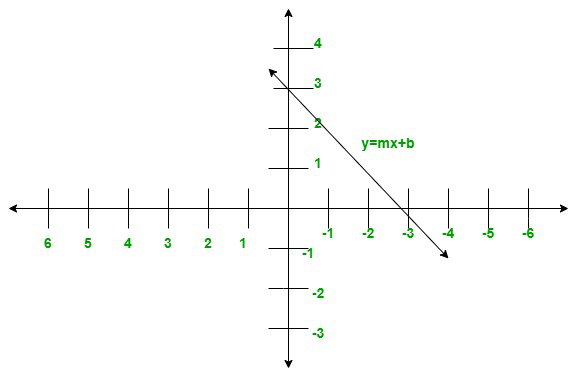### Graph formula

The graph formula or the slope-intercept form for the straight-line equation gives the relation between two points with respect to which a line is plotted on the graph. The formula or the equation we receive as a solution makes the plotting of graphs convenient.

The graph formula is derived from the two points of the line. Let the two points be (a1, b1) and (a2, b2), and the graph formula or slope-intercept form of the straight line will be given by,

y = mx + b

Where,

m is the slope

And, b is the y-intercept

In the graph formulas, the slope (m) is also determined by the given points. And, the slope is determined by the slope formula.

### Slope

A slope is a slanted line segment or steepness of a line that is plotted on a graph. The slope is determined by the slope formula by the ratio of values of y-intercept and x-intercept.

Mathematically,

Slope (m) = (b2 – b1)/(a2 – a1)

Hence, a graph is plotted by the equation formed by the relation of the slope of the line and y-intercept.

### Sample Problems

Question 1: Form an equation of a line with a slope of 3 and a y-intercept of 12.

Solution:

Given,

The slope (m) is 3/2

The y-intercept is 4

Now,

y = mx + b

y = 3/2 × x + 4

y = 3/2 x + 4

Hence, the required equation is 3/2x + 4.

The graph of the given equation is plotted below: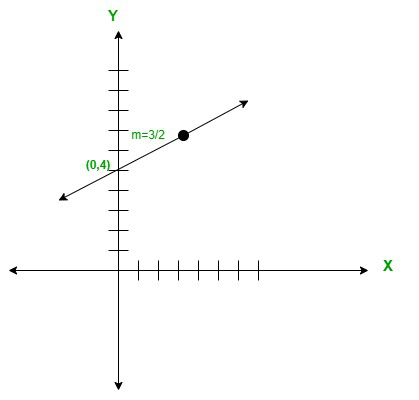Question 2: Form an equation of a line with a slope -6 and y-intercept of 15.

Solution:

Given,

The slope (m) is -4/3

The y-intercept is 10

Now,

y = mx + b

y = (-4/3) × x + 10

y = -4/3x + 10

Hence, the required equation is y = -4/3x + 10.

The graph of the given equation is plotted below: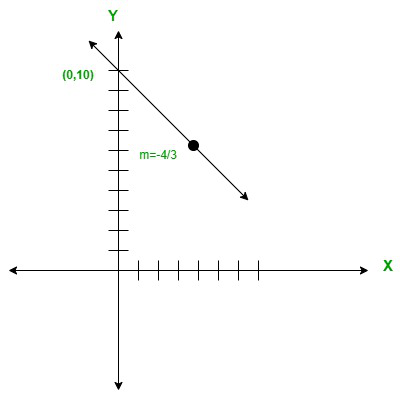Question 3: Form an equation of a line with a slope of 2 and y-intercept of 13.

Solution:

Given,

The slope (m) is 2.

The y-intercept is -7.

Now,

y = mx + b

y = 2 × x + (-7)

y = 2x -7

Hence, the required equation is y=2x-7.

The graph of the given equation is plotted below: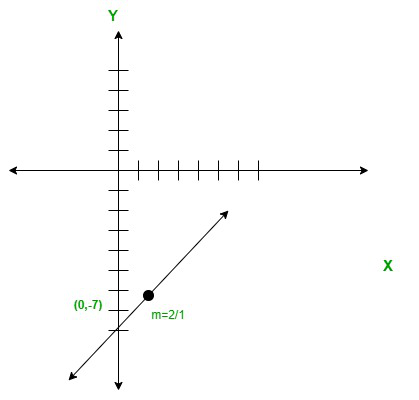Question 4: Form an equation of a line with a slope of 3 and passes through (0, -5).

Solution:

Given,

The slope (m) is 3/2

The point (x, y) = (0, -5)

Now,

y = mx + b

y = 3/2 × (0) + b

y = b

Here,

y-intercept, b = -5

Therefore, The equation will be given by,

y = mx + b

y = 3/2x – 5

Hence, the required equation is y = 3/2x – 5.

The graph of the given equation is plotted below: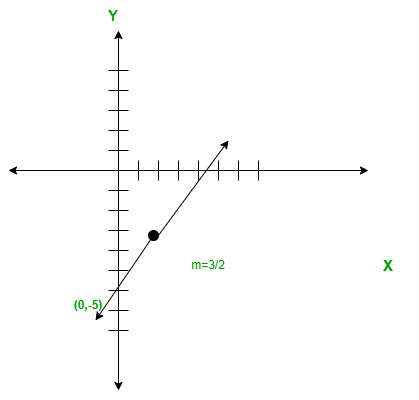Question 5: Form an equation of a line with a slope of 6 and a y-intercept of 25.

Solution:

Given,

The slope (m) is 6/5

The y-intercept is 5.

Now,

y = mx + b

y = -6/5 × x + 5

y = -6/5x + 5

Hence, the require equation is -6/5x + 5.

The graph of the given equation is plotted below: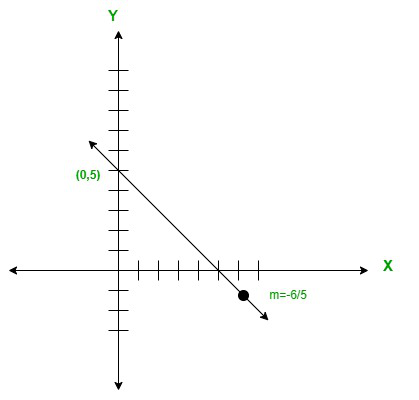Question 6: Form an equation of a line with a slope of 4/3 and passes through (0, -7).

Solution:

Given,

The slope (m) is 4/3

The point (x, y) = (0,-7)

Now,

y = mx + b

y = 4/3 × (0) + b

y = b

Here,

y-intercept, b = -7

Therefore, The equation will be given by,

y = mx + b

y = 4/3x – 7

Hence, the required equation is y = 4/3x – 7.

The graph of the given equation is plotted below: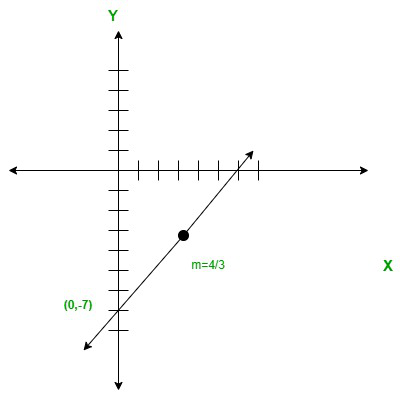My Personal Notes arrow_drop_up
Recommended Articles
Page :